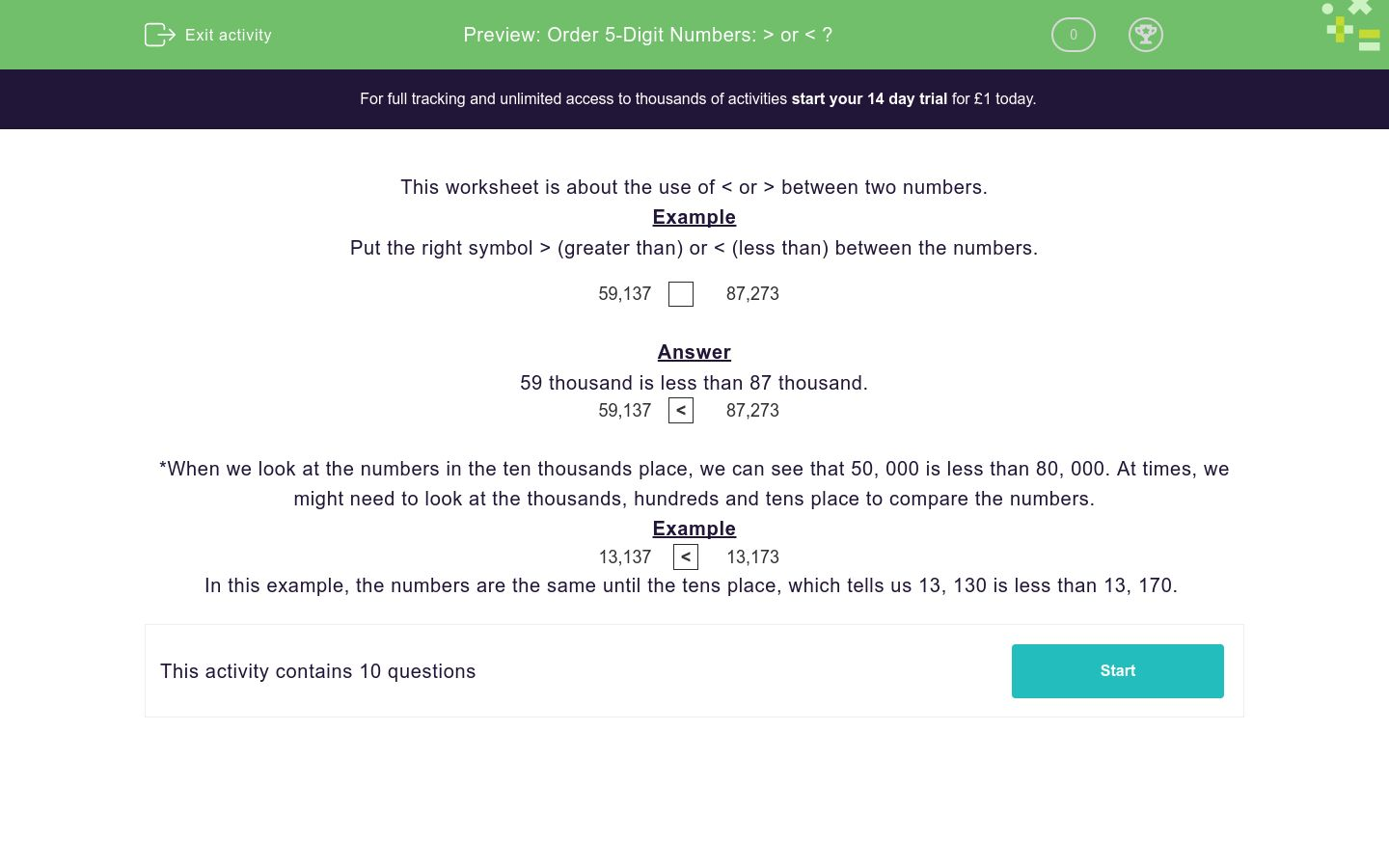# Order 5-Digit Numbers: > or < ?

In this worksheet, students use the symbols > and < correctly between two large numbers.Key stage:  KS 2

Curriculum topic:   Number: Number and Place Value

Curriculum subtopic:   Know Numbers to 1 000 000

Difficulty level:### QUESTION 1 of 10

This worksheet is about the use of < or > between two numbers.

Example

Put the right symbol > (greater than) or < (less than) between the numbers.

59,137    87,273

59 thousand is less than 87 thousand.

59,137
 <
87,273

*When we look at the numbers in the ten thousands place, we can see that 50, 000 is less than 80, 000. At times, we might need to look at the thousands, hundreds and tens place to compare the numbers.

Example

13,137
 <
13,173

In this example, the numbers are the same until the tens place, which tells us 13, 130 is less than 13, 170.

Put the right symbol > (greater than) or < (less than) between the numbers.

32,006    91,873
<

>

Put the right symbol > (greater than) or < (less than) between the numbers.

92,563    32,287
<

>

Put the right symbol > (greater than) or < (less than) between the numbers.

10,159    20,335
<

>

Put the right symbol > (greater than) or < (less than) between the numbers.

60,974    95,509

<

>

Put the right symbol > (greater than) or < (less than) between the numbers.

70,251    13,255

<

>

Put the right symbol > (greater than) or < (less than) between the numbers.

25,556    84,782

<

>

Put the right symbol > (greater than) or < (less than) between the numbers.

85,673    92,389

<

>

Put the right symbol > (greater than) or < (less than) between the numbers.

83,132    47,328

<

>

Put the right symbol > (greater than) or < (less than) between the numbers.

22,124    22,770

<

>

Put the right symbol > (greater than) or < (less than) between the numbers.

59,137    59,173

<

>

• Question 1

Put the right symbol > (greater than) or < (less than) between the numbers.

32,006    91,873
<
EDDIE SAYS
32, 006 < 91, 873 30, 000 is less than 90, 000.
• Question 2

Put the right symbol > (greater than) or < (less than) between the numbers.

92,563    32,287
>
EDDIE SAYS
92, 563 > 32, 287 90, 000 is greater than 30, 000.
• Question 3

Put the right symbol > (greater than) or < (less than) between the numbers.

10,159    20,335
<
EDDIE SAYS
10, 159 < 20, 335 10, 000 is less than 20, 000.
• Question 4

Put the right symbol > (greater than) or < (less than) between the numbers.

60,974    95,509

<
EDDIE SAYS
60, 974 < 95, 509 60, 000 is less than 90, 000.
• Question 5

Put the right symbol > (greater than) or < (less than) between the numbers.

70,251    13,255

>
EDDIE SAYS
70, 251 > 13, 255 70, 000 is greater than 13, 000.
• Question 6

Put the right symbol > (greater than) or < (less than) between the numbers.

25,556    84,782

<
EDDIE SAYS
25, 556 < 84, 782 20, 000 is less than 80, 000.
• Question 7

Put the right symbol > (greater than) or < (less than) between the numbers.

85,673    92,389

<
EDDIE SAYS
85, 673 < 92, 389 80, 000 is less than 90, 000.
• Question 8

Put the right symbol > (greater than) or < (less than) between the numbers.

83,132    47,328

>
EDDIE SAYS
83, 132 > 47, 328 80, 000 is greater than 40, 000.
• Question 9

Put the right symbol > (greater than) or < (less than) between the numbers.

22,124    22,770

<
EDDIE SAYS
22, 124 < 22, 770 22, 100 is less than 22, 700.
• Question 10

Put the right symbol > (greater than) or < (less than) between the numbers.

59,137    59,173

<
EDDIE SAYS
59, 137 < 59, 173 59, 130 is less than 59, 170.
---- OR ----

Sign up for a £1 trial so you can track and measure your child's progress on this activity.

### What is EdPlace?

We're your National Curriculum aligned online education content provider helping each child succeed in English, maths and science from year 1 to GCSE. With an EdPlace account you’ll be able to track and measure progress, helping each child achieve their best. We build confidence and attainment by personalising each child’s learning at a level that suits them.

Get started